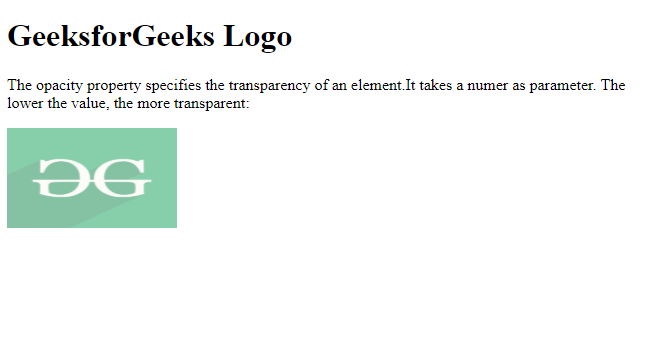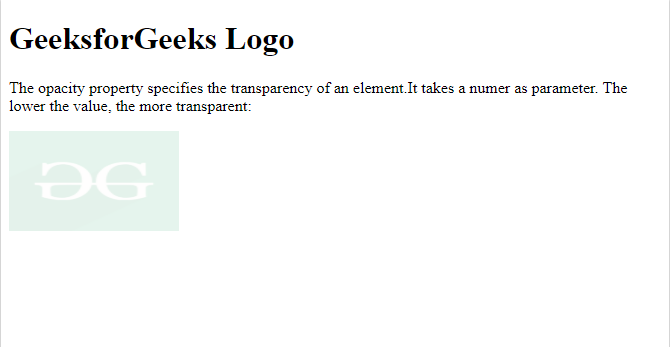Open in App
Not now

# CSS | Number Data Type

• Last Updated : 24 Aug, 2022

CSS Value is represented by <number> which takes an integer as a parameter or a number with a fractional component. They can be used by the symbol (+) or (-). The number can be positive or negative. All the digits of the number are 0 to 9 and all are of numeric types.

Syntax:

`<number>`

Note: No unit associated with numbers.

Examples for valid numbers

`23, +90, -76, 0.56, 34.67, 10e4, -4.6e-2`

Examples for invalid numbers

`23., -+45, 45.7.6`

Example 1: The following example demonstrates applying opacity to any element.

## HTML

 `` `<``html``>` `<``head``>` `    ``<``style``>` `        ``img {` `            ``opacity: 0.5;` `        ``}` `    ``` `` `<``body``>` `    ``<``h1``>GeeksforGeeks Logo` `    ``<``p``>` `        ``The opacity property specifies ` `        ``the transparency of an element.` `        ``It takes a number as parameter.` `        ``The lower the value, the image ` `        ``is more transparent ` `    ``` `    ``<``img` `src=` `"https://media.geeksforgeeks.org/wp-content/cdn-uploads/20190710102234/download3.png"` `        ``alt="GFG" width="170" height="100">` `` ``

Output:Example 2: The following example shows the opacity property with some changed value.

## HTML

 `` `<``html``>` `<``head``>` `    ``<``style``>` `        ``img {` `            ``opacity: .11;` `        ``}` `    ``` `` `<``body``>` `    ``<``h1``>GeeksforGeeks Logo]` `    ``<``p``>` `        ``The opacity property specifies ` `        ``the transparency of an element.` `        ``It takes a number as parameter.` `        ``The lower the value, the image ` `        ``is more transparent:` `    ``` `    ``<``img` `src=` `"https://media.geeksforgeeks.org/wp-content/cdn-uploads/20190710102234/download3.png"` `        ``alt="GFG" width="170" height="100">` `` ``

Output:Supported Browsers:

• Chrome 1
• Edge 12
• Firefox 1
• Opera 2
• Safar 1

My Personal Notes arrow_drop_up
Related Articles